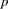Introduction to Categorical Data Analysis Procedures

# Comparison of PROC FREQ and the Modeling Procedures

PROC FREQ is used primarily to investigate the relationship between two variables; any confounding variables are taken into account by stratification rather than by parameter estimation. Modeling procedures are used to investigate the relationship among many variables, all of which are integrated into a parametric model.

When a modeling procedure estimates the covariance matrix of the frequencies, it assumes that the frequencies were obtained by a stratified simple random-sampling procedure. However, some modeling procedures can handle different sampling methods. PROC CATMOD can analyze input data that consists of a function vector and a covariance matrix, so you can estimate the covariance matrix of the frequencies in the appropriate manner before modeling the data. PROC SURVEYLOGISTIC can analyze data from a completely different, but known, sampling scheme.

For the FREQ procedure, Fisher’s Exact Test and Cochran-Mantel-Haenszel (CMH) statistics are based on the hypergeometric distribution, which corresponds to fixed marginal totals. However, by conditioning arguments, these tests are generally applicable to a wide range of sampling procedures. Similarly, the Pearson and likelihood-ratio chi-square statistics can be derived under a variety of sampling situations.

PROC FREQ can do some traditional nonparametric analysis (such as the Kruskal-Wallis test and Spearman’s correlation) since it can generate rank scores internally. Fisher’s Exact Test and the CMH statistics are also inherently nonparametric. However, the main vehicle for nonparametric analyses in the SAS System is the NPAR1WAY procedure.

A large sample size is required for the validity of the chi-square distributions, the standard errors, and the covariance matrices for PROC FREQ and the modeling procedures. If sample size is a problem, then PROC FREQ has the advantage with its CMH statistics because it does not use any degrees of freedom to estimate parameters for confounding variables. In addition, PROC FREQ can compute exact-values for any two-way table, provided that the sample size is sufficiently small in relation to the size of the table. It can also produce exact-values for many tests, including the test of binomial proportions, the Cochran-Armitage test for trend, and the Jonckheere-Terpstra test for ordered differences among classes. PROC LOGISTIC can perform exact conditional logistic regression and Firth’s penalized-likelihood regression to compensate for small sample sizes.

See the procedure chapters for more information. In addition, some well-known texts that deal with analyzing categorical data are listed in the "References" section of this chapter.Previous Page | Next Page | Top of Page